# Hardware Design Details - 2023.2 English

## Vitis Tutorials: AI Engine

Document ID
XD100
Release Date
2023-11-29
Version
2023.2 English
Matrix Multiplication using DSP58 Implementation Architecture

### Matrix Multiplication using DSP58 Implementation Architecture

In this design, Matrix Multiplication is implemented using DSP58 Systolic array of size 32x32. i.e There are 32 DSP58 cascade chains, each chain having 32 DSP58s. Thus 32x32 matrix is the basic matrix multiplication size. Larger matrices are broken down into submatrices of size 32x32.

Basic 32x32 Multiplication is performed as follows -

Matrix A row data moves upwards along DSP A Port cascade chain. For first 32 clocks data is only shifted into the DSP chains. So after 32 clocks, Row 0 of Matrix A is populated in first DSP cascade chain, Row 1 is populated in next cascade chain and so on, as shown in the below diagram. show in the below diagram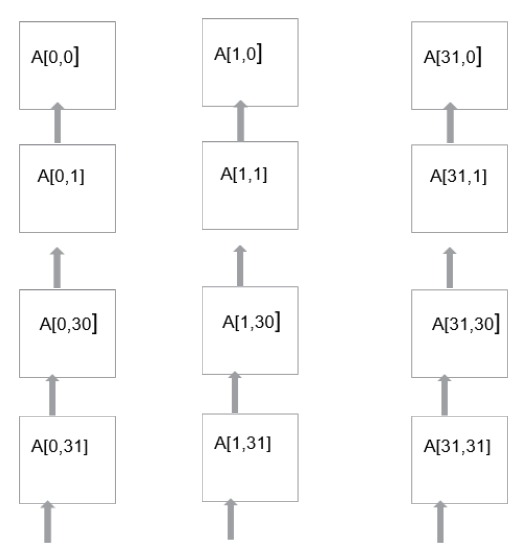#### Calculating First Row of Output Matrix

After Matrix A elements are shifted into cascade chain, last row of matrix B is driven clock-by-clock to the bottom most DSP of the first cascade chain, as shown in the below diagram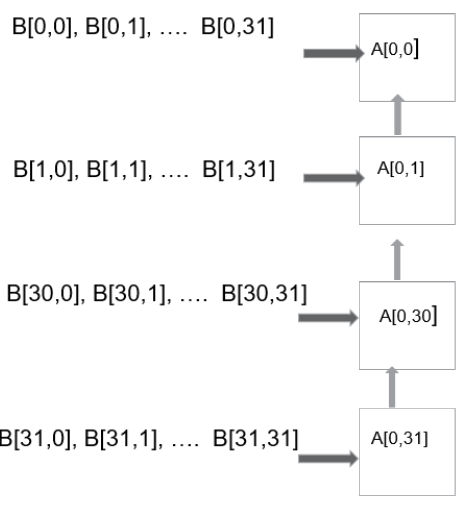First Row of output matrix is calculated as follows -

Bottom most DSP calculates A[0,31] B[31,0] and sends the output to upper DSP via PCOUT cascade port. On 2nd clock upper DSP starts receiving B[30,0], B[30,1], … B[30,31] (i.e Row 30 of Matrix B). So,on 2nd clock, 2nd DSP calculates A[0,30] B [30,0] + PCOUT = A[0,30] B[30,0] + A[0,31] B[31,0], and sends it up to the 3rd DSP. 3rd DSP starts receiving Matrix B Column 29 on 3rd clock, computes 3rd MAC operation and send up to 4th DSP. Thus after 32nd clock, top DSP has generated Row 0 Column 0 element of the output matrix.

On 2nd clock, bottom DSP receives B[31,1] and it calculates A[0,31] * B[31,1] which is the beginning of the MAC operation for Row 0 Column 1 element of output matrix. Row 0, Column 1 calculations traverse upwards in a similar way, and on 33rd clock, top DSP generates Row 0 Column 1 element of the output matrix.

Similarly for next 30 clocks, (that is, clock 34 to 63) top DSP of first cascade chain generates other 30 elements of Row 0 of the output matrix

Other rows of output matrix are calculated as follows -

B[31,0], B[31,1], … B[31,31] elements, that is row 31 of Matrix B is shifted to next DSP chain every clock. Hence Start of driving Matrix A Rows to subsequent DSP chains is also started with one clock delay. So bottom DSP of 2nd cascade chain starts on 2nd clock and it computes A[1,31] * B[31,0], which is beginning of the MAC operation for Row 1 Column 0 element of output matrix. Thus 2nd cascade chain is 1 clock delayed wrt first cascade chain and it generates its 32 outputs from clock 33 to 64. These outputs are Row 1 of the output matrix. Each subsequenct cascade chain is one clock delayed wrt previous chain, and thus the last cascade chain generates Row 31 outputs on clock 63 to 94.

#### 32x32 Matrix Multiplication Latency

For the first 32 clocks, Matrix A Row 0 is loaded into first cascade chain. Over next 32 clocks, First cascade chain calculates first row of output matrix, and for next 32 clocks, other rows of output matrix are generated. However after 64 clocks, first DSP cascade chain can receive first row data for next 32x32 matrix.

Larger matrices are broken down into smaller 32x32 matrices. For example, 1Kx1Kx1K Matrices are represented as follows, where each box is 32x32 matrix –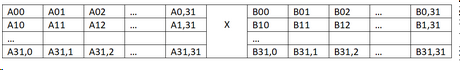Output matrix is -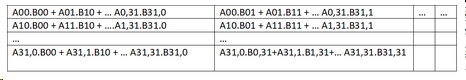#### Data Flow for larger matrices

Matrix A00 is first multiplied with Matrix B00, which is the basic 32x32 matrix multiplication. Over the first 96 clocks, each DSP chain produces 32 outputs, thus total 1K outputs are generated which are the partial sums for the final output. These partial sums are written to 64 partial sum BRAMs. After 64 clocks, first cascade chain is done with A00 B00 submatrix, and it then starts performing A00 B01 to calculate partial sums for the next column of the output matrix. Likewise over next 32 clocks, other DSP cascade chains will also complete A00 B00 matrix multiplication and move to A00 B01 submatrix multiplication. This way Matrix A00 is multiplied with Matrix B00, B01, B02 … B0,31.

This completes A00 submatrix multiplications. Next, we read A01 submatrix of Matrix A, and it gets multiplied with submatrices of Matrix B. The partial sums are added to the partial sums previous generated, and stored back. Thus we will keep moving along the first row of Matirx A and multiply that submatrix with submatrices of Matirx B. This will continue for 32 iterations, and in the 32nd iteration, data is written to Output BRAM instead of partial Sum BRAM. This completes computation of the first row of the output matrix.

Then we will move to the next row of Matrix A and all these steps are repeated. After 32 such iterations, 1Kx1Kx1K matrix multiplication will be completed.

#### Matrix Calculation Latency for large matrices

32x32 matrix calculation requires 96 clocks. However first cascade chain in the DSP58 array is done with its computation after 64 clocks, and it can start receiving data for next submatrix. Thus for 32 clocks, there is overlap of previous and new submatrix calculations. So the total number of clocks required for large matix multiplication is 64 * No. of Sbumatrices + 32.

In this design, DSP clock is operating at 750MHz (1.33ns).

The following figure shows block diagram of the design.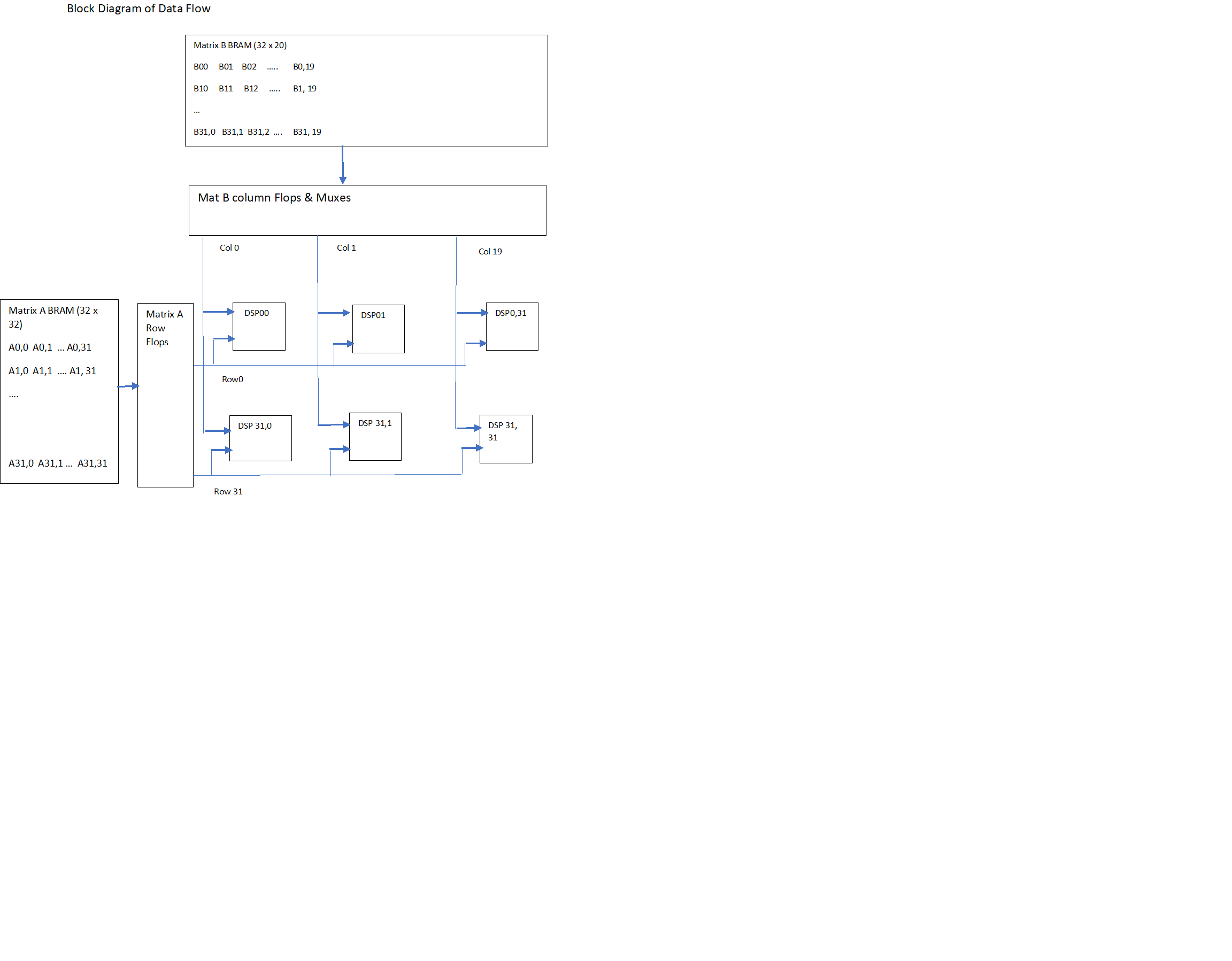PL Kernel Details